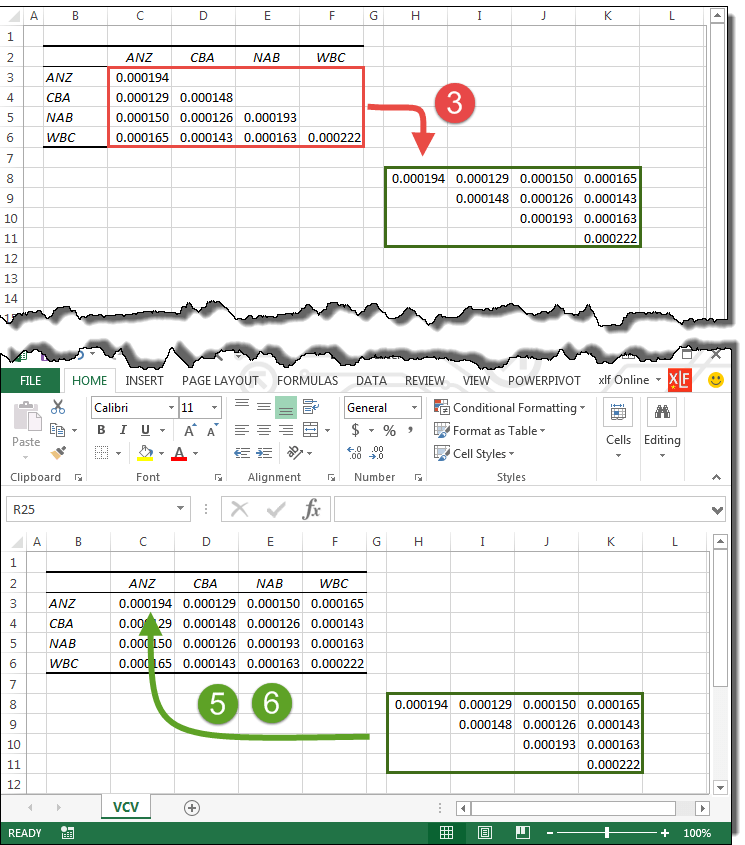# Convert a lower triangular table to a full matrix [using Copy → Paste Special]

This module demonstrates how to convert the lower triangular covariance table from the Excel Analysis ToolPak to a full covariance matrix for use with the MMULT function.

First, a brief review of square matrices.

A matrix is a rectangular array of numbers of the form $$A=\begin{bmatrix} a_{11} & \cdots & a_{1n} \\ \vdots & \ddots & \vdots \\ a_{m1} & \cdots & a_{mn} \end{bmatrix}$$ and is called an m x n matrix because it has m rows and n columns. Matrix $A$ can also be written as $A=(a_{ij} )$ where $a_{ij}$ is an element of $A$ in i-th row and j-th column where $1 \leq i \leq m$ and $1 \leq j \leq n$. The diagonal elements are $(a_{ij} ) \; \forall \; i=j$.

## Square matrices

$$B=\begin{bmatrix} 5 & -2 & 6 \\ -2 & 11 & 9.1 \\ 6 & 9.1 & 10 \end{bmatrix}$$

The matrix $B$ is a 3 x 3 square matrix because it has equal numbers of rows and columns, that is, $m = n = 3$. The diagonal elements are the values $5, 11, 10$

### 1. Upper triangular matrix

The $n \times n$ matrix $C$, is upper triangular if all elements below the main diagonal are 0. $$C_1=\begin{bmatrix} 5 & 2 & 6 & 7 \\ 0 & 11 & 9 & 4 \\ 0 & 0 & 10 & 1 \\ 0 & 0 & 0 & 8\end{bmatrix}, \qquad C_2=\begin{bmatrix} 5 & 2 & 6 & 7 \\ 0 & 0 & 0 & 4 \\ 0 & 0 & 0 & 1 \\ 0 & 0 & 0 & 8\end{bmatrix}$$

### 2. Lower triangular matrix

The $n \times n$ matrix $C$, is lower triangular if all elements above the main diagonal are 0. $$C_3=\begin{bmatrix} 5 & 0 & 0 & 0 \\ 3 & 9 & 0 & 0 \\ 7 & 2 & 1 & 0 \\ 4 & 5 & 8 & 6\end{bmatrix}, \qquad C_4=\begin{bmatrix} 5 & 0 & 0 & 0 \\ 3 & 0 & 0 & 0 \\ 7 & 4 & 4 & 0 \\ 6 & 9 & 1 & 8\end{bmatrix}$$

### 3. Diagonal matrix

The $n \times n$ matrix $C$, is diagonal if all elements off the main diagonal are 0. $$C_5=\begin{bmatrix} 5 & 0 & 0 & 0 \\ 0 & 9 & 0 & 0 \\ 0 & 0 & 1 & 0 \\ 0 & 0 & 0 & 6\end{bmatrix}, \qquad C_6=\begin{bmatrix} 5 & 0 & 0 & 0 \\ 0 & 0 & 0 & 0 \\ 0 & 0 & 4 & 0 \\ 0 & 0 & 0 & 8\end{bmatrix}$$ $C_5$ has diagonal elements $5,9,1,6$. $C_6$ has diagonal elements $5,0,4,8$.

### 4. Symmetrical matrix

The $n \times n$ matrix $C$, is symmetrical if the matrix is equal to its transpose. That is, $C=C^T$ $$C_7=\begin{bmatrix} 5 & 3 & 7 & 4 \\ 3 & 9 & 2 & 5 \\ 7 & 2 & 1 & 8 \\ 4 & 5 & 8 & 6\end{bmatrix}$$

## Lower triangular covariance table

The Analysis ToolPak includes tools to estimate Covariance, and Correlation. Both procedures produce output that is lower triangular. The omission of the upper triangle was originally based on the need to save several bytes of (expensive) computer memory. The same reason the Toolpak being a Add-In and activated only when required. An example of the Covariance table for four stock returns is shown in figure 1.Fig 1: Lower triangular covariance table: ToolPak output B2:F6 (top panel), full matrix B2:F6 (lower panel). The circle numbers 3, 5, and 6 refers to the step numbers listed below

It is clear from figure 1, however, that the output is not a lower triangular matrix, as described in point 2 above, because the upper triangle is blank rather contain zeros. The output is better described as a lower triangular table.

To convert the lower triangular table to a symmetrical matrix for use in an MMULT equation, do the following:

1. Select the 4 x 4 lower triangular variance-covariance array (with the red frame in figure 1), and
2. Copy the Selection to the Clipboard
3. Select the top left cell of a temporary work area (cell H8) and Paste > Special > Transpose. You can also select Paste > Values to avoid format issues
4. Select the transposed array from step 3 (with the green frame in figure 1), and copy to the Clipboard
5. Select the top left cell, C3 of the variance-covariance array from step 1, then
6. Paste > Special > Skip Blanks

• This example was developed in Excel 2013 Pro 64 bit.

• Published: 20 April 2015
• Revised: Wednesday 12th of February 2020 - 03:58 PM, [Australian Eastern Standard Time (EST)]